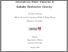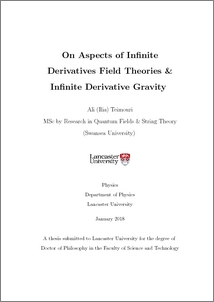# On aspects of infinite derivatives field theories & infinite derivative gravity

Teimouri, Ilia and Gratus, Jonathan (2018) On aspects of infinite derivatives field theories & infinite derivative gravity. PhD thesis, Lancaster University.Preview
PDF (2018teimouriphd)
2018teimouriphd.pdf - Published Version

## Abstract

Infinite derivative theory of gravity is a modification to the general theory of relativity. Such modification maintains the massless graviton as the only true physical degree of freedom and avoids ghosts. Moreover, this class of modified gravity can address classical singularities. In this thesis some essential aspects of an infinite derivative theory of gravity are studied. Namely, we considered the Hamiltonian formalism, where the true physical degrees of freedom for infinite derivative scalar models and infinite derivative gravity are obtained. Furthermore, the Gibbons-Hawking-York boundary term for the infinite derivative theory of gravity was obtained. Finally, we considered the thermodynamical aspects of the infinite derivative theory of gravity over different backgrounds. Throughout the thesis, our methodology is applied to general relativity, Gauss-Bonnet and f(R) theories of gravity as a check and validation.

Item Type:
Thesis (PhD)
Departments:
ID Code:
90105
Deposited By:
Deposited On:
01 Feb 2018 10:32
Refereed?:
No
Published?:
Published